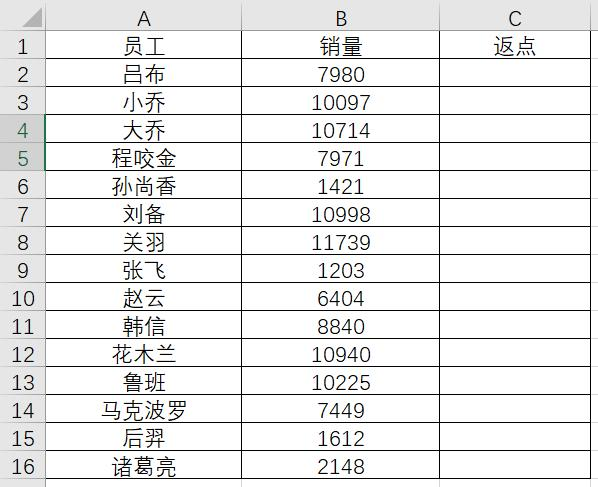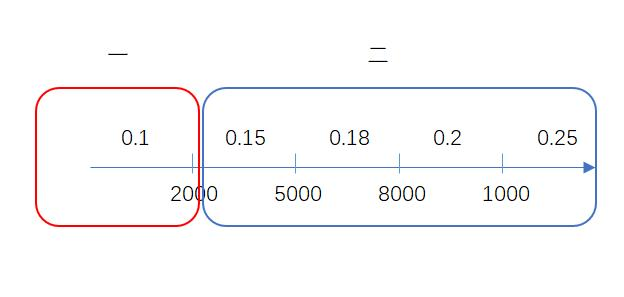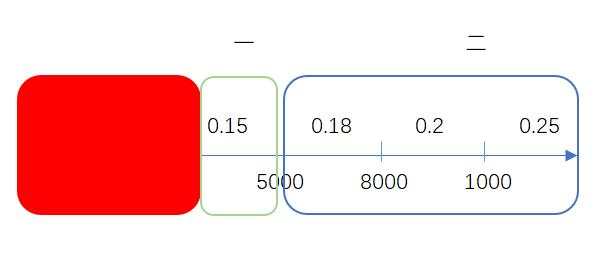X2的判断是，IF(B2<8000,0.18,X3)

X3的判断是，IF(B2<10000,0.2,X4)

X4的值为0.25

=IF(B2<2000,0.1,IF(B2<5000,0.18,IF(B2<8000,0.2,IF(B2<1000,0.2,0.25))))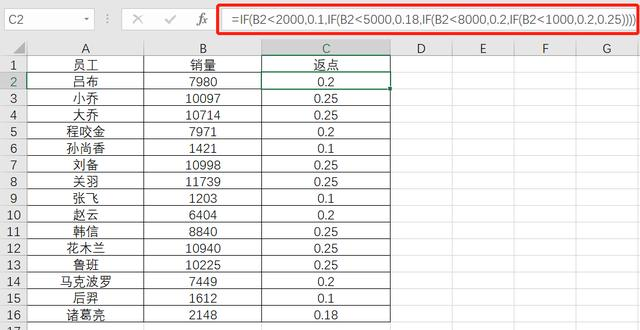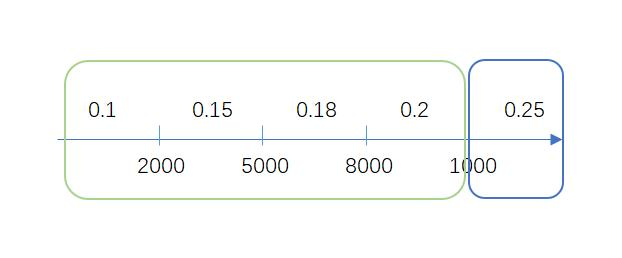X2是IF(B2<8000,X3,0.2)IF(B2>10000,0.25,X1)

X1为IF(B2>8000,0.2,X2)

....依次递推，得到最终的公式结果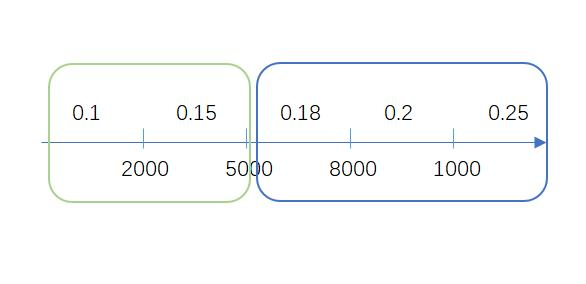--------------------------